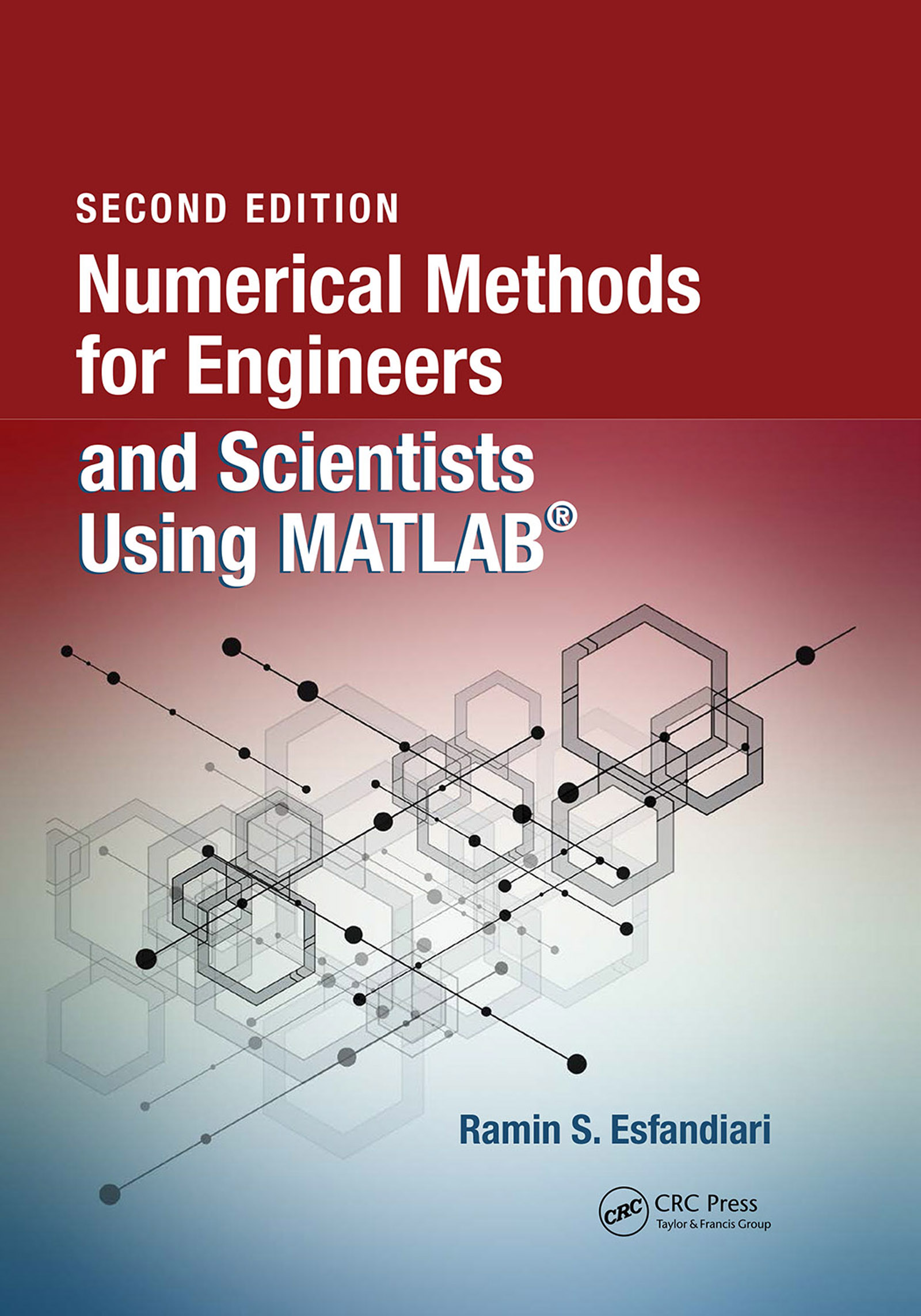# Numerical Methods for Engineers and Scientists Using MATLAB®

## 2nd Edition

CRC Press

471 pages | 109 Color Illus. | 4 B/W Illus.

##### Purchasing Options:\$ = USD
Hardback: 9781498777421
pub: 2017-03-20
SAVE ~\$32.00
\$160.00
\$128.00
x
eBook (VitalSource) : 9781315152417
pub: 2017-04-25
from \$80.00

FREE Standard Shipping!

### Description

This book provides a pragmatic, methodical and easy-to-follow presentation of numerical methods and their effective implementation using MATLAB, which is introduced at the outset. The author introduces techniques for solving equations of a single variable and systems of equations, followed by curve fitting and interpolation of data. The book also provides detailed coverage of numerical differentiation and integration, as well as numerical solutions of initial-value and boundary-value problems. The author then presents the numerical solution of the matrix eigenvalue problem, which entails approximation of a few or all eigenvalues of a matrix. The last chapter is devoted to numerical solutions of partial differential equations that arise in engineering and science. Each method is accompanied by at least one fully worked-out example showing essential details involved in preliminary hand calculations, as well as computations in MATLAB. This thoroughly-researched resource:

### Table of Contents

Background and Introduction. Introduction to MATLAB®. Solution of Equations of a Single Variable. Solution of Systems of Equations. Curve Fitting (Approximation) and Interpolation. Curve Fitting (Approximation) and Interpolation. Numerical Differentiation and Integration. Numerical Solution of Initial-Value Problems. Numerical Solution of Boundary-Value Problems. Matrix Eigenvalue Problem. Numerical Solution of Partial Differential Equations. Bibliography. Index.

### About the Author

Dr. Ramin Esfandiari is a professor of Mechanical and Aerospace Engineering at California State University, Long Beach (CSULB), where he has served as a faculty member since 1989. He received his B.S. in Mechanical Engineering, and M.A. and Ph.D. in Applied Mathematics (Optimal Control), all from the University of California, Santa Barbara.

He has authored several refereed research papers in high quality engineering and scientific journals, and more than 10 books, including Modeling and Analysis of Dynamic Systems, 2nd Edition (CRC Press, 2014, with Dr. Bei Lu) and Applied Mathematics for Engineers, 5th Edition (Atlantis, 2013).

Professor Esfandiari is the recipient of several teaching and research awards, including two Meritorious Performance and Professional Promise Awards, TRW Excellence in Teaching and Scholarship Award, and the Distinguished Faculty Teaching Award.

### Subject Categories

##### BISAC Subject Codes/Headings:
MAT003000
MATHEMATICS / Applied
MAT021000
MATHEMATICS / Number Systems
TEC009070
TECHNOLOGY & ENGINEERING / Mechanical